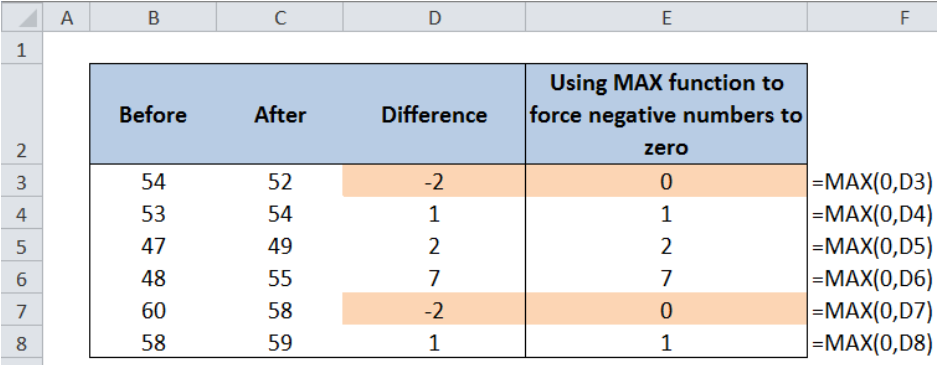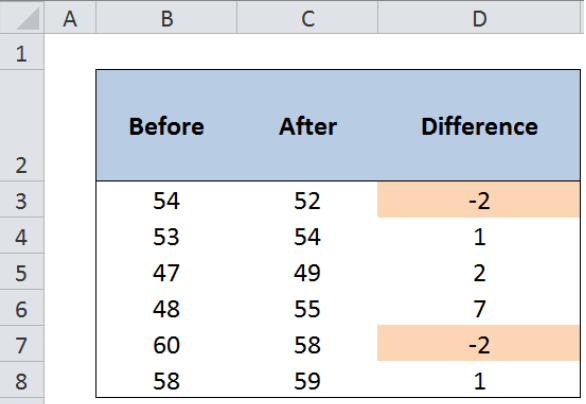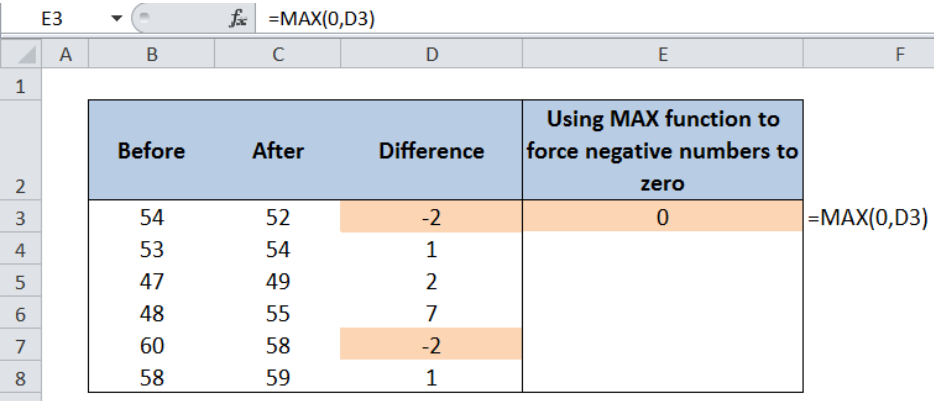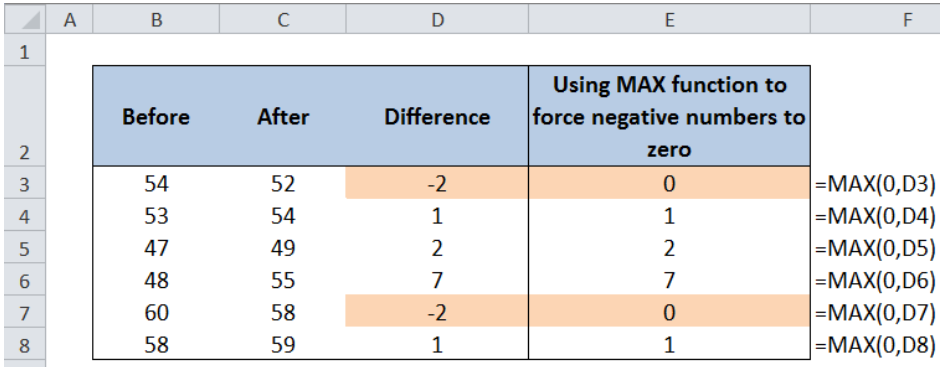Get instant live expert help with Excel or Google Sheets“My Excelchat expert helped me in less than 20 minutes, saving me what would have been 5 hours of work!”

#### Post your problem and you’ll get Expert help in seconds.

Your message must be at least 40 characters
Our professional Expert are available now. Your privacy is guaranteed.

# Force negative numbers to zero

There is a simple method in Excel that forces negative numbers to zero through the use of the MAX function. This tutorial will walk through how to do it.Figure 1. Final result: Forcing negative numbers to zero using MAX function

## Setting up the Data

Below is a table of the Before and After data, with corresponding differences.  We want to force the negative numbers in column D to zero and retain the positive numbers.Figure 2.  Sample data for forcing negative numbers to zero

The quickest way to force negative numbers to zero is by using the MAX function in Excel.

## MAX function in Excel

The MAX function returns the largest value in a set of values.

### Syntax

`=MAX(number1, number 2,...)`

In cell E3, enter the formula:

`=MAX(0,D3)`Figure 3.  Entering the MAX formula

• This formula compares the number zero “0” and the value in D3, then returns the larger value
• Zero is larger than any negative number; in this case, zero is larger than -2
• The MAX function returns 0 in cell E3

Copy the formula in the succeeding rows. Below table shows how the MAX function has forced the negative numbers to zero in cells E3 and E7.Figure 4.   Final result: Forcing negative numbers to zero using MAX function

Most of the time, the problem you will need to solve will be more complex than a simple application of a formula or function. If you want to save hours of research and frustration, try our live Excelchat service! Our Excel Experts are available 24/7 to answer any Excel question you may have. We guarantee a connection within 30 seconds and a customized solution within 20 minutes.

Are you still looking for help with the IF function? View our comprehensive round-up of IF function tutorials here.

### Did this post not answer your question? Get a solution from connecting with the expert.Another blog reader asked this question today on Excelchat:
Solution examplesI want a formula (or combination of formulas) to look up the matching values(full name) within one column and based on those matching look in 2nd and 3rd column (email in and email out in last 6mo) and indicate which one is the most/highest. If there is a tie, then look in 4th and 5th column (email in and out in last year). It could be indicated by a yes or number to say that that name.
Solved by E. H. in 40 minsI need a formula to calculate the MAX of a range of 167 rows in column D, then find the MAX of the next 167 rows in column D and so on continuously through the entire sheet. Example MAX D2:D169, D170:D337, D338:D505 etc...
Solved by I. Q. in 60 minsHi, i need one formula, for example in salary level 200000 to 300000 is coming under 6B 300000 to 450000 is coming under 6A 450000 to 600000 is coming under 5A Now if salary is 280000 so which formula i can use to find their Band lavel
Solved by E. F. in 35 minsI have a list of cost prices in one column and i would like to know what formula to use to add a percentage value. E.g if my cost price is just Â£1 i would like to create the sell price to be perhaps Â£3, but if my cost price is Â£1000, i would like the sell price to perhaps be Â£1,100 (not Â£3,000).
Solved by M. Y. in 60 minsIf I have a sheet that pulls data from another sheet. How can I compare if the right data is filled in, after the fact?
Solved by Z. Y. in 43 mins## Subscribe to Excelchat.coAnother blog reader asked this question today on Excelchat: